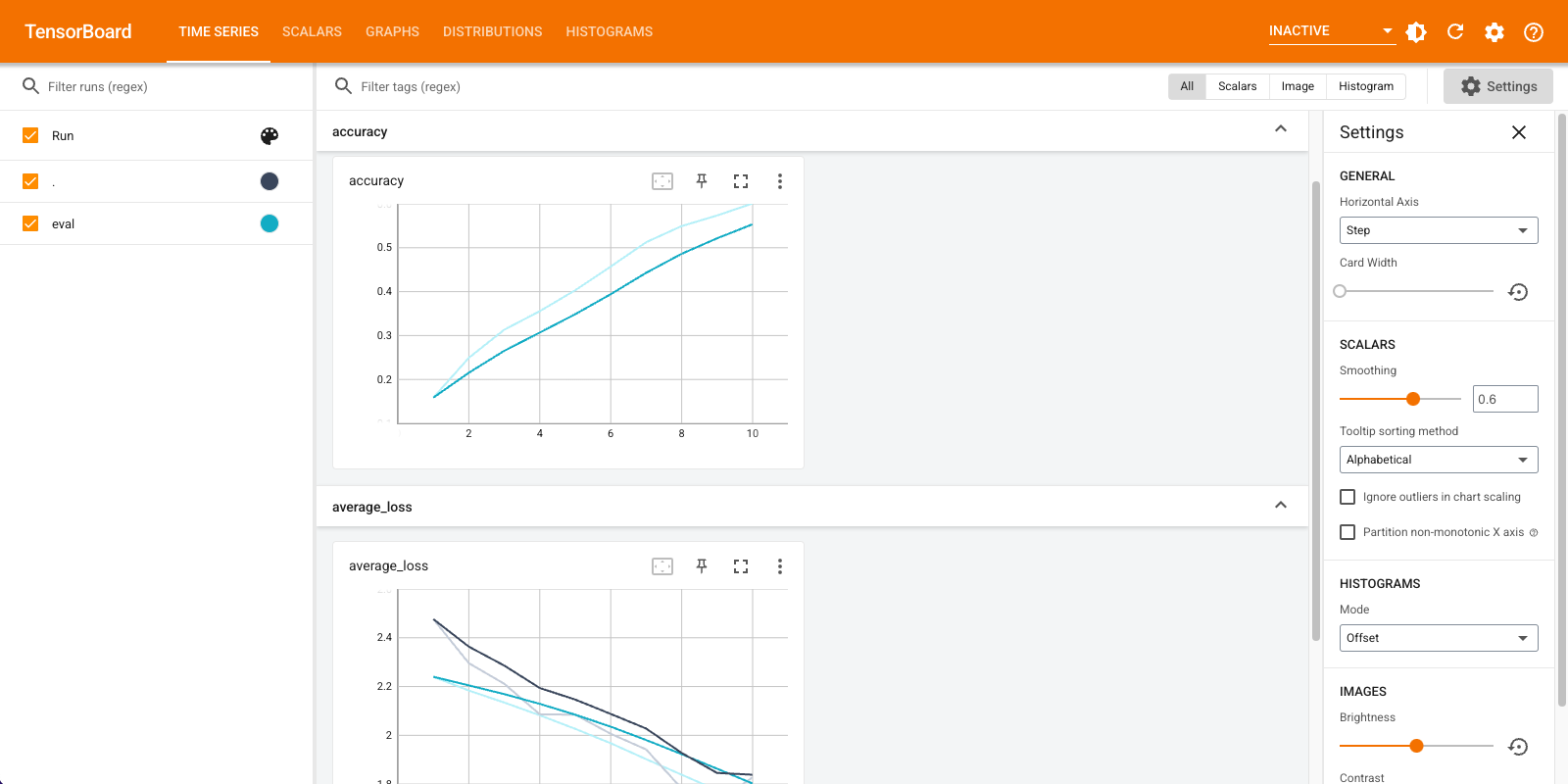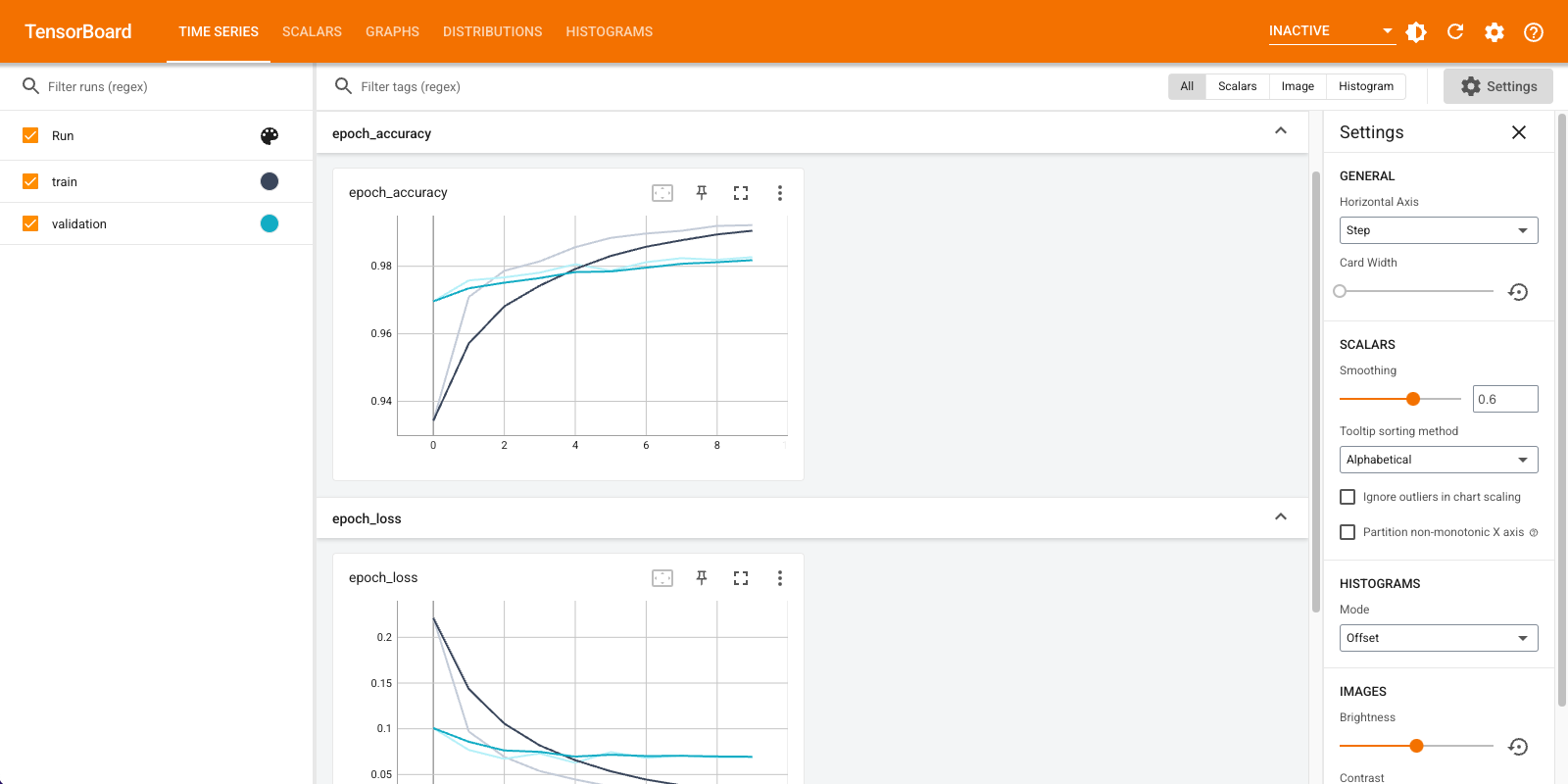# Migrate TensorBoard: TensorFlow's visualization toolkit

TensorBoard is a built-in tool for providing measurements and visualizations in TensorFlow. Common machine learning experiment metrics, such as accuracy and loss, can be tracked and displayed in TensorBoard. TensorBoard is compatible with TensorFlow 1 and 2 code.

In TensorFlow 1, `tf.estimator.Estimator` saves summaries for TensorBoard by default. In comparison, in TensorFlow 2, summaries can be saved using a `tf.keras.callbacks.TensorBoard` callback.

This guide demonstrates how to use TensorBoard, first, in TensorFlow 1 with Estimators, and then, how to carry out the equivalent process in TensorFlow 2.

### Setup

``````import tensorflow.compat.v1 as tf1
import tensorflow as tf
import tempfile
import numpy as np
import datetime
``````
``````mnist = tf.keras.datasets.mnist # The MNIST dataset.

x_train, x_test = x_train / 255.0, x_test / 255.0
``````
```Downloading data from https://storage.googleapis.com/tensorflow/tf-keras-datasets/mnist.npz
11493376/11490434 [==============================] - 0s 0us/step
11501568/11490434 [==============================] - 0s 0us/step
```

### TensorFlow 1: TensorBoard with tf.estimator

In this TensorFlow 1 example, you instantiate a `tf.estimator.DNNClassifier`, train and evaluate it on the MNIST dataset, and use TensorBoard to display the metrics:

``````%reload_ext tensorboard

feature_columns = [tf1.feature_column.numeric_column("x", shape=[28, 28])]

config = tf1.estimator.RunConfig(save_summary_steps=1,
save_checkpoints_steps=1)

path = tempfile.mkdtemp()

classifier = tf1.estimator.DNNClassifier(
feature_columns=feature_columns,
hidden_units=[256, 32],
n_classes=10,
dropout=0.1,
model_dir=path,
config = config
)

train_input_fn = tf1.estimator.inputs.numpy_input_fn(
x={"x": x_train},
y=y_train.astype(np.int32),
num_epochs=10,
batch_size=50,
shuffle=True,
)

test_input_fn = tf1.estimator.inputs.numpy_input_fn(
x={"x": x_test},
y=y_test.astype(np.int32),
num_epochs=10,
shuffle=False
)

train_spec = tf1.estimator.TrainSpec(input_fn=train_input_fn, max_steps=10)
eval_spec = tf1.estimator.EvalSpec(input_fn=test_input_fn,
steps=10,
throttle_secs=0)

tf1.estimator.train_and_evaluate(estimator=classifier,
train_spec=train_spec,
eval_spec=eval_spec)
``````
```INFO:tensorflow:Using config: {'_model_dir': '/tmp/tmp9w0s5sgg', '_tf_random_seed': None, '_save_summary_steps': 1, '_save_checkpoints_steps': 1, '_save_checkpoints_secs': None, '_session_config': allow_soft_placement: true
graph_options {
rewrite_options {
meta_optimizer_iterations: ONE
}
}
, '_keep_checkpoint_max': 5, '_keep_checkpoint_every_n_hours': 10000, '_log_step_count_steps': 100, '_train_distribute': None, '_device_fn': None, '_protocol': None, '_eval_distribute': None, '_experimental_distribute': None, '_experimental_max_worker_delay_secs': None, '_session_creation_timeout_secs': 7200, '_checkpoint_save_graph_def': True, '_service': None, '_cluster_spec': ClusterSpec({}), '_task_type': 'worker', '_task_id': 0, '_global_id_in_cluster': 0, '_master': '', '_evaluation_master': '', '_is_chief': True, '_num_ps_replicas': 0, '_num_worker_replicas': 1}

INFO:tensorflow:Not using Distribute Coordinator.
INFO:tensorflow:Running training and evaluation locally (non-distributed).
INFO:tensorflow:Start train and evaluate loop. The evaluate will happen after every checkpoint. Checkpoint frequency is determined based on RunConfig arguments: save_checkpoints_steps 1 or save_checkpoints_secs None.
WARNING:tensorflow:From /tmpfs/src/tf_docs_env/lib/python3.7/site-packages/tensorflow/python/training/training_util.py:236: Variable.initialized_value (from tensorflow.python.ops.variables) is deprecated and will be removed in a future version.
Instructions for updating:
Use Variable.read_value. Variables in 2.X are initialized automatically both in eager and graph (inside tf.defun) contexts.
WARNING:tensorflow:From /tmpfs/src/tf_docs_env/lib/python3.7/site-packages/tensorflow_estimator/python/estimator/inputs/queues/feeding_queue_runner.py:65: QueueRunner.__init__ (from tensorflow.python.training.queue_runner_impl) is deprecated and will be removed in a future version.
Instructions for updating:
To construct input pipelines, use the `tf.data` module.
WARNING:tensorflow:From /tmpfs/src/tf_docs_env/lib/python3.7/site-packages/tensorflow_estimator/python/estimator/inputs/queues/feeding_functions.py:491: add_queue_runner (from tensorflow.python.training.queue_runner_impl) is deprecated and will be removed in a future version.
Instructions for updating:
To construct input pipelines, use the `tf.data` module.
INFO:tensorflow:Calling model_fn.
INFO:tensorflow:Done calling model_fn.
INFO:tensorflow:Create CheckpointSaverHook.
INFO:tensorflow:Graph was finalized.
INFO:tensorflow:Running local_init_op.
INFO:tensorflow:Done running local_init_op.
WARNING:tensorflow:From /tmpfs/src/tf_docs_env/lib/python3.7/site-packages/tensorflow/python/training/monitored_session.py:907: start_queue_runners (from tensorflow.python.training.queue_runner_impl) is deprecated and will be removed in a future version.
Instructions for updating:
To construct input pipelines, use the `tf.data` module.
INFO:tensorflow:Calling checkpoint listeners before saving checkpoint 0...
INFO:tensorflow:Saving checkpoints for 0 into /tmp/tmp9w0s5sgg/model.ckpt.
INFO:tensorflow:Calling checkpoint listeners after saving checkpoint 0...
INFO:tensorflow:Calling checkpoint listeners before saving checkpoint 1...
INFO:tensorflow:Saving checkpoints for 1 into /tmp/tmp9w0s5sgg/model.ckpt.
INFO:tensorflow:Calling checkpoint listeners after saving checkpoint 1...
INFO:tensorflow:Calling model_fn.
INFO:tensorflow:Done calling model_fn.
INFO:tensorflow:Starting evaluation at 2021-09-22T20:09:45
INFO:tensorflow:Graph was finalized.
INFO:tensorflow:Restoring parameters from /tmp/tmp9w0s5sgg/model.ckpt-1
INFO:tensorflow:Running local_init_op.
INFO:tensorflow:Done running local_init_op.
INFO:tensorflow:Evaluation [1/10]
INFO:tensorflow:Evaluation [2/10]
INFO:tensorflow:Evaluation [3/10]
INFO:tensorflow:Evaluation [4/10]
INFO:tensorflow:Evaluation [5/10]
INFO:tensorflow:Evaluation [6/10]
INFO:tensorflow:Evaluation [7/10]
INFO:tensorflow:Evaluation [8/10]
INFO:tensorflow:Evaluation [9/10]
INFO:tensorflow:Evaluation [10/10]
INFO:tensorflow:Inference Time : 0.25105s
INFO:tensorflow:Finished evaluation at 2021-09-22-20:09:45
INFO:tensorflow:Saving dict for global step 1: accuracy = 0.16328125, average_loss = 2.2459474, global_step = 1, loss = 287.48126
INFO:tensorflow:Saving 'checkpoint_path' summary for global step 1: /tmp/tmp9w0s5sgg/model.ckpt-1
INFO:tensorflow:loss = 115.65201, step = 0
INFO:tensorflow:Calling checkpoint listeners before saving checkpoint 2...
INFO:tensorflow:Saving checkpoints for 2 into /tmp/tmp9w0s5sgg/model.ckpt.
INFO:tensorflow:Calling checkpoint listeners after saving checkpoint 2...
INFO:tensorflow:Calling model_fn.
INFO:tensorflow:Done calling model_fn.
INFO:tensorflow:Starting evaluation at 2021-09-22T20:09:46
INFO:tensorflow:Graph was finalized.
INFO:tensorflow:Restoring parameters from /tmp/tmp9w0s5sgg/model.ckpt-2
INFO:tensorflow:Running local_init_op.
INFO:tensorflow:Done running local_init_op.
INFO:tensorflow:Evaluation [1/10]
INFO:tensorflow:Evaluation [2/10]
INFO:tensorflow:Evaluation [3/10]
INFO:tensorflow:Evaluation [4/10]
INFO:tensorflow:Evaluation [5/10]
INFO:tensorflow:Evaluation [6/10]
INFO:tensorflow:Evaluation [7/10]
INFO:tensorflow:Evaluation [8/10]
INFO:tensorflow:Evaluation [9/10]
INFO:tensorflow:Evaluation [10/10]
INFO:tensorflow:Inference Time : 0.24542s
INFO:tensorflow:Finished evaluation at 2021-09-22-20:09:46
INFO:tensorflow:Saving dict for global step 2: accuracy = 0.25625, average_loss = 2.1821105, global_step = 2, loss = 279.31015
INFO:tensorflow:Saving 'checkpoint_path' summary for global step 2: /tmp/tmp9w0s5sgg/model.ckpt-2
INFO:tensorflow:Calling checkpoint listeners before saving checkpoint 3...
INFO:tensorflow:Saving checkpoints for 3 into /tmp/tmp9w0s5sgg/model.ckpt.
INFO:tensorflow:Calling checkpoint listeners after saving checkpoint 3...
INFO:tensorflow:Calling model_fn.
INFO:tensorflow:Done calling model_fn.
INFO:tensorflow:Starting evaluation at 2021-09-22T20:09:46
INFO:tensorflow:Graph was finalized.
INFO:tensorflow:Restoring parameters from /tmp/tmp9w0s5sgg/model.ckpt-3
INFO:tensorflow:Running local_init_op.
INFO:tensorflow:Done running local_init_op.
INFO:tensorflow:Evaluation [1/10]
INFO:tensorflow:Evaluation [2/10]
INFO:tensorflow:Evaluation [3/10]
INFO:tensorflow:Evaluation [4/10]
INFO:tensorflow:Evaluation [5/10]
INFO:tensorflow:Evaluation [6/10]
INFO:tensorflow:Evaluation [7/10]
INFO:tensorflow:Evaluation [8/10]
INFO:tensorflow:Evaluation [9/10]
INFO:tensorflow:Evaluation [10/10]
INFO:tensorflow:Inference Time : 0.24425s
INFO:tensorflow:Finished evaluation at 2021-09-22-20:09:46
INFO:tensorflow:Saving dict for global step 3: accuracy = 0.3, average_loss = 2.1269565, global_step = 3, loss = 272.25043
INFO:tensorflow:Saving 'checkpoint_path' summary for global step 3: /tmp/tmp9w0s5sgg/model.ckpt-3
INFO:tensorflow:Calling checkpoint listeners before saving checkpoint 4...
INFO:tensorflow:Saving checkpoints for 4 into /tmp/tmp9w0s5sgg/model.ckpt.
INFO:tensorflow:Calling checkpoint listeners after saving checkpoint 4...
INFO:tensorflow:Calling model_fn.
INFO:tensorflow:Done calling model_fn.
INFO:tensorflow:Starting evaluation at 2021-09-22T20:09:47
INFO:tensorflow:Graph was finalized.
INFO:tensorflow:Restoring parameters from /tmp/tmp9w0s5sgg/model.ckpt-4
INFO:tensorflow:Running local_init_op.
INFO:tensorflow:Done running local_init_op.
INFO:tensorflow:Evaluation [1/10]
INFO:tensorflow:Evaluation [2/10]
INFO:tensorflow:Evaluation [3/10]
INFO:tensorflow:Evaluation [4/10]
INFO:tensorflow:Evaluation [5/10]
INFO:tensorflow:Evaluation [6/10]
INFO:tensorflow:Evaluation [7/10]
INFO:tensorflow:Evaluation [8/10]
INFO:tensorflow:Evaluation [9/10]
INFO:tensorflow:Evaluation [10/10]
INFO:tensorflow:Inference Time : 0.25865s
INFO:tensorflow:Finished evaluation at 2021-09-22-20:09:47
INFO:tensorflow:Saving dict for global step 4: accuracy = 0.3484375, average_loss = 2.0719538, global_step = 4, loss = 265.21008
INFO:tensorflow:Saving 'checkpoint_path' summary for global step 4: /tmp/tmp9w0s5sgg/model.ckpt-4
INFO:tensorflow:Calling checkpoint listeners before saving checkpoint 5...
INFO:tensorflow:Saving checkpoints for 5 into /tmp/tmp9w0s5sgg/model.ckpt.
WARNING:tensorflow:From /tmpfs/src/tf_docs_env/lib/python3.7/site-packages/tensorflow/python/training/saver.py:971: remove_checkpoint (from tensorflow.python.training.checkpoint_management) is deprecated and will be removed in a future version.
Instructions for updating:
Use standard file APIs to delete files with this prefix.
INFO:tensorflow:Calling checkpoint listeners after saving checkpoint 5...
INFO:tensorflow:Calling model_fn.
INFO:tensorflow:Done calling model_fn.
INFO:tensorflow:Starting evaluation at 2021-09-22T20:09:47
INFO:tensorflow:Graph was finalized.
INFO:tensorflow:Restoring parameters from /tmp/tmp9w0s5sgg/model.ckpt-5
INFO:tensorflow:Running local_init_op.
INFO:tensorflow:Done running local_init_op.
INFO:tensorflow:Evaluation [1/10]
INFO:tensorflow:Evaluation [2/10]
INFO:tensorflow:Evaluation [3/10]
INFO:tensorflow:Evaluation [4/10]
INFO:tensorflow:Evaluation [5/10]
INFO:tensorflow:Evaluation [6/10]
INFO:tensorflow:Evaluation [7/10]
INFO:tensorflow:Evaluation [8/10]
INFO:tensorflow:Evaluation [9/10]
INFO:tensorflow:Evaluation [10/10]
INFO:tensorflow:Inference Time : 0.23830s
INFO:tensorflow:Finished evaluation at 2021-09-22-20:09:47
INFO:tensorflow:Saving dict for global step 5: accuracy = 0.40078124, average_loss = 2.013233, global_step = 5, loss = 257.69382
INFO:tensorflow:Saving 'checkpoint_path' summary for global step 5: /tmp/tmp9w0s5sgg/model.ckpt-5
INFO:tensorflow:Calling checkpoint listeners before saving checkpoint 6...
INFO:tensorflow:Saving checkpoints for 6 into /tmp/tmp9w0s5sgg/model.ckpt.
INFO:tensorflow:Calling checkpoint listeners after saving checkpoint 6...
INFO:tensorflow:Calling model_fn.
INFO:tensorflow:Done calling model_fn.
INFO:tensorflow:Starting evaluation at 2021-09-22T20:09:48
INFO:tensorflow:Graph was finalized.
INFO:tensorflow:Restoring parameters from /tmp/tmp9w0s5sgg/model.ckpt-6
INFO:tensorflow:Running local_init_op.
INFO:tensorflow:Done running local_init_op.
INFO:tensorflow:Evaluation [1/10]
INFO:tensorflow:Evaluation [2/10]
INFO:tensorflow:Evaluation [3/10]
INFO:tensorflow:Evaluation [4/10]
INFO:tensorflow:Evaluation [5/10]
INFO:tensorflow:Evaluation [6/10]
INFO:tensorflow:Evaluation [7/10]
INFO:tensorflow:Evaluation [8/10]
INFO:tensorflow:Evaluation [9/10]
INFO:tensorflow:Evaluation [10/10]
INFO:tensorflow:Inference Time : 0.24491s
INFO:tensorflow:Finished evaluation at 2021-09-22-20:09:48
INFO:tensorflow:Saving dict for global step 6: accuracy = 0.45546874, average_loss = 1.9495137, global_step = 6, loss = 249.53775
INFO:tensorflow:Saving 'checkpoint_path' summary for global step 6: /tmp/tmp9w0s5sgg/model.ckpt-6
INFO:tensorflow:Calling checkpoint listeners before saving checkpoint 7...
INFO:tensorflow:Saving checkpoints for 7 into /tmp/tmp9w0s5sgg/model.ckpt.
INFO:tensorflow:Calling checkpoint listeners after saving checkpoint 7...
INFO:tensorflow:Calling model_fn.
INFO:tensorflow:Done calling model_fn.
INFO:tensorflow:Starting evaluation at 2021-09-22T20:09:48
INFO:tensorflow:Graph was finalized.
INFO:tensorflow:Restoring parameters from /tmp/tmp9w0s5sgg/model.ckpt-7
INFO:tensorflow:Running local_init_op.
INFO:tensorflow:Done running local_init_op.
INFO:tensorflow:Evaluation [1/10]
INFO:tensorflow:Evaluation [2/10]
INFO:tensorflow:Evaluation [3/10]
INFO:tensorflow:Evaluation [4/10]
INFO:tensorflow:Evaluation [5/10]
INFO:tensorflow:Evaluation [6/10]
INFO:tensorflow:Evaluation [7/10]
INFO:tensorflow:Evaluation [8/10]
INFO:tensorflow:Evaluation [9/10]
INFO:tensorflow:Evaluation [10/10]
INFO:tensorflow:Inference Time : 0.24294s
INFO:tensorflow:Finished evaluation at 2021-09-22-20:09:49
INFO:tensorflow:Saving dict for global step 7: accuracy = 0.4984375, average_loss = 1.8863195, global_step = 7, loss = 241.4489
INFO:tensorflow:Saving 'checkpoint_path' summary for global step 7: /tmp/tmp9w0s5sgg/model.ckpt-7
INFO:tensorflow:Calling checkpoint listeners before saving checkpoint 8...
INFO:tensorflow:Saving checkpoints for 8 into /tmp/tmp9w0s5sgg/model.ckpt.
INFO:tensorflow:Calling checkpoint listeners after saving checkpoint 8...
INFO:tensorflow:Calling model_fn.
INFO:tensorflow:Done calling model_fn.
INFO:tensorflow:Starting evaluation at 2021-09-22T20:09:49
INFO:tensorflow:Graph was finalized.
INFO:tensorflow:Restoring parameters from /tmp/tmp9w0s5sgg/model.ckpt-8
INFO:tensorflow:Running local_init_op.
INFO:tensorflow:Done running local_init_op.
INFO:tensorflow:Evaluation [1/10]
INFO:tensorflow:Evaluation [2/10]
INFO:tensorflow:Evaluation [3/10]
INFO:tensorflow:Evaluation [4/10]
INFO:tensorflow:Evaluation [5/10]
INFO:tensorflow:Evaluation [6/10]
INFO:tensorflow:Evaluation [7/10]
INFO:tensorflow:Evaluation [8/10]
INFO:tensorflow:Evaluation [9/10]
INFO:tensorflow:Evaluation [10/10]
INFO:tensorflow:Inference Time : 0.24205s
INFO:tensorflow:Finished evaluation at 2021-09-22-20:09:49
INFO:tensorflow:Saving dict for global step 8: accuracy = 0.50390625, average_loss = 1.8260235, global_step = 8, loss = 233.731
INFO:tensorflow:Saving 'checkpoint_path' summary for global step 8: /tmp/tmp9w0s5sgg/model.ckpt-8
INFO:tensorflow:Calling checkpoint listeners before saving checkpoint 9...
INFO:tensorflow:Saving checkpoints for 9 into /tmp/tmp9w0s5sgg/model.ckpt.
INFO:tensorflow:Calling checkpoint listeners after saving checkpoint 9...
INFO:tensorflow:Calling model_fn.
INFO:tensorflow:Done calling model_fn.
INFO:tensorflow:Starting evaluation at 2021-09-22T20:09:49
INFO:tensorflow:Graph was finalized.
INFO:tensorflow:Restoring parameters from /tmp/tmp9w0s5sgg/model.ckpt-9
INFO:tensorflow:Running local_init_op.
INFO:tensorflow:Done running local_init_op.
INFO:tensorflow:Evaluation [1/10]
INFO:tensorflow:Evaluation [2/10]
INFO:tensorflow:Evaluation [3/10]
INFO:tensorflow:Evaluation [4/10]
INFO:tensorflow:Evaluation [5/10]
INFO:tensorflow:Evaluation [6/10]
INFO:tensorflow:Evaluation [7/10]
INFO:tensorflow:Evaluation [8/10]
INFO:tensorflow:Evaluation [9/10]
INFO:tensorflow:Evaluation [10/10]
INFO:tensorflow:Inference Time : 0.24289s
INFO:tensorflow:Finished evaluation at 2021-09-22-20:09:50
INFO:tensorflow:Saving dict for global step 9: accuracy = 0.5046875, average_loss = 1.770147, global_step = 9, loss = 226.57881
INFO:tensorflow:Saving 'checkpoint_path' summary for global step 9: /tmp/tmp9w0s5sgg/model.ckpt-9
INFO:tensorflow:Calling checkpoint listeners before saving checkpoint 10...
INFO:tensorflow:Saving checkpoints for 10 into /tmp/tmp9w0s5sgg/model.ckpt.
INFO:tensorflow:Calling checkpoint listeners after saving checkpoint 10...
INFO:tensorflow:Calling model_fn.
INFO:tensorflow:Done calling model_fn.
INFO:tensorflow:Starting evaluation at 2021-09-22T20:09:50
INFO:tensorflow:Graph was finalized.
INFO:tensorflow:Restoring parameters from /tmp/tmp9w0s5sgg/model.ckpt-10
INFO:tensorflow:Running local_init_op.
INFO:tensorflow:Done running local_init_op.
INFO:tensorflow:Evaluation [1/10]
INFO:tensorflow:Evaluation [2/10]
INFO:tensorflow:Evaluation [3/10]
INFO:tensorflow:Evaluation [4/10]
INFO:tensorflow:Evaluation [5/10]
INFO:tensorflow:Evaluation [6/10]
INFO:tensorflow:Evaluation [7/10]
INFO:tensorflow:Evaluation [8/10]
INFO:tensorflow:Evaluation [9/10]
INFO:tensorflow:Evaluation [10/10]
INFO:tensorflow:Inference Time : 0.24503s
INFO:tensorflow:Finished evaluation at 2021-09-22-20:09:50
INFO:tensorflow:Saving dict for global step 10: accuracy = 0.50859374, average_loss = 1.7165858, global_step = 10, loss = 219.72298
INFO:tensorflow:Saving 'checkpoint_path' summary for global step 10: /tmp/tmp9w0s5sgg/model.ckpt-10
INFO:tensorflow:Loss for final step: 82.50392.
({'accuracy': 0.50859374,
'average_loss': 1.7165858,
'loss': 219.72298,
'global_step': 10},
[])
```
``````%tensorboard --logdir {classifier.model_dir}
``````### TensorFlow 2: TensorBoard with a Keras callback and Model.fit

In this TensorFlow 2 example, you create and store logs with the `tf.keras.callbacks.TensorBoard` callback, and train the model. The callback tracks the accuracy and loss per epoch. It is passed to `Model.fit` in the `callbacks` list.

``````%reload_ext tensorboard

def create_model():
return tf.keras.models.Sequential([
tf.keras.layers.Flatten(input_shape=(28, 28)),
tf.keras.layers.Dense(512, activation='relu'),
tf.keras.layers.Dropout(0.2),
tf.keras.layers.Dense(10, activation='softmax')
])

model = create_model()
loss='sparse_categorical_crossentropy',
metrics=['accuracy'],
steps_per_execution=10)

log_dir = tempfile.mkdtemp()
tensorboard_callback = tf.keras.callbacks.TensorBoard(
log_dir=log_dir,
histogram_freq=1) # Enable histogram computation with each epoch.

model.fit(x=x_train,
y=y_train,
epochs=10,
validation_data=(x_test, y_test),
callbacks=[tensorboard_callback])
``````
```Epoch 1/10
1875/1875 [==============================] - 4s 2ms/step - loss: 0.2205 - accuracy: 0.9351 - val_loss: 0.1078 - val_accuracy: 0.9675
Epoch 2/10
1875/1875 [==============================] - 3s 2ms/step - loss: 0.0958 - accuracy: 0.9711 - val_loss: 0.0852 - val_accuracy: 0.9716
Epoch 3/10
1875/1875 [==============================] - 3s 2ms/step - loss: 0.0685 - accuracy: 0.9786 - val_loss: 0.0728 - val_accuracy: 0.9772
Epoch 4/10
1875/1875 [==============================] - 3s 2ms/step - loss: 0.0525 - accuracy: 0.9834 - val_loss: 0.0694 - val_accuracy: 0.9786
Epoch 5/10
1875/1875 [==============================] - 3s 1ms/step - loss: 0.0429 - accuracy: 0.9860 - val_loss: 0.0705 - val_accuracy: 0.9784
Epoch 6/10
1875/1875 [==============================] - 3s 2ms/step - loss: 0.0369 - accuracy: 0.9876 - val_loss: 0.0616 - val_accuracy: 0.9822
Epoch 7/10
1875/1875 [==============================] - 3s 1ms/step - loss: 0.0316 - accuracy: 0.9897 - val_loss: 0.0777 - val_accuracy: 0.9787
Epoch 8/10
1875/1875 [==============================] - 3s 2ms/step - loss: 0.0267 - accuracy: 0.9910 - val_loss: 0.0709 - val_accuracy: 0.9806
Epoch 9/10
1875/1875 [==============================] - 3s 2ms/step - loss: 0.0239 - accuracy: 0.9918 - val_loss: 0.0651 - val_accuracy: 0.9835
Epoch 10/10
1875/1875 [==============================] - 3s 2ms/step - loss: 0.0225 - accuracy: 0.9920 - val_loss: 0.0713 - val_accuracy: 0.9819
<keras.callbacks.History at 0x7f3b2c590310>
```
``````%tensorboard --logdir {tensorboard_callback.log_dir}
``````[]
[]